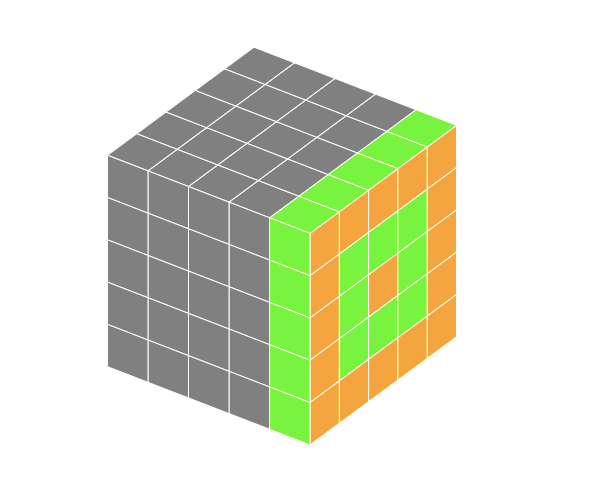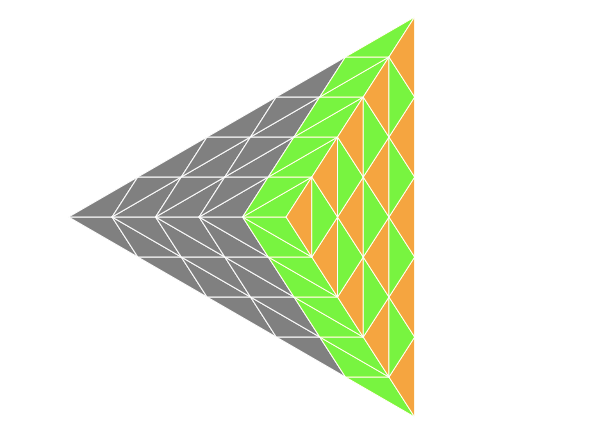medium.com Go back Open original

# Creating 3D twisty puzzles using programming

You must be familiar with the standard 3x3x3 Rubik’s cube, in which the goal is to solve the scrambled puzzle so as to match all the stickers on each of the faces. In this post, we’ll take a look at how we can create whole families of such twisty puzzles — things as complex as Rubik’s cubes or face turning Octahedra of arbitrary sizes using simple problem-solving techniques.

Note that instead of a comprehensive step by step tutorial, this article will focus on conveying the core ideas that you can use to play with for your own projects. There will be some questions I will leave open-ended & some details I will skip. Feel free to contact me & check the source if you wish to dig deeper. Follow up articles on this are a possibility as well :)

Now, since this is a complex problem, it makes sense to break it down into simpler tasks that we can tackle one by one.
To create a twisty puzzle, we basically need to do the following 3 things:

1. Compose the shape.
2. Slice the shape into pieces.
3. Stitch the pieces to form cycles.

Let’s start with defining the simplest 3D shape, Cube. If we had a real solid object, we would not perceive most of what constitutes it, we would only see the surface. While creating a virtual version of it, the only approach that makes sense is to model only the surfaces.

The surface of a cube is composed of 6 square faces, attached to 8 vertices. Using just lists of numbers, it can be defined like this:

`// list of 8 corner points as (x, y, z) tripletspoints = [[1,1,1],[1,1,-1],…,[-1,-1,-1]]`
`// list of 6 faces (4 indices each referring to the point list)faces = [[0,1,3,2],[0,1,5,4],…,[4,5,7,6]]`

Now we need to render the cube. The simplest approach for it is performing parallel projection after depth sort i.e. sort the faces by z-coordinates & render them on (x, y) plane by simply ignoring the z-coordinate values of the points.

For the cube we just defined, only one of the square faces will be visible after projection. To change the view, we need to alter the orientation of the cube. For this, we can perform two 2D rotations, one by angle `θ` around the z-axis & another by angle `ϕ`around the x-axis. To rotate a point around origin on a plane, we can use the following equations:

`x' = x cos(α) - y sin(α)y' = x sin(α) + y cos(α)`

Here, `x`& `y` can be replaced by the coordinates that are affected by the rotation. Note that although you do not need to understand where these equations come from for using them, I would highly recommend studying a little about linear transformations to develop a feel for why it works.

We can alter the angles θ & ϕ according to cursor movements so that user can re-orient the object however s/he wants. We can also set up constant angular velocities for both of these parameters & update these angular distances in a game loop, which will result in nice rotating animations.

The embedded codepen implements this using HTML5 Canvas API

As an exercise, the following problems can be played with before moving on to the next task:

1. How can we compose other platonic solids using these ideas?
2. How can we link cursor movements to the object orientation?
3. Is there any better way to orient a 3D shape according to user input?

When you look at our model of the cube, Slicing the faces into stickers appears to be equivalent to adding more information to the system. There will be more independent objects, the collection of grid points that serves as the skeleton will be much larger.

This is where Object-Oriented Programming starts to become more important for us. Although not necessary for computation, OOP techniques can make the code much more manageable for a human when there is a lot of information flowing around.

Let’s define a few classes before we begin slicing,

1. Point: Locations in 3D space.
2. Vector: Movements in 3D space.
3. Sticker: Composed of point objects ordered by right-hand thumb rule.
4. Face: Collection of sticker objects.
5. Puzzle: Composed of face objects.

The vector class can be used to define methods for operations such as vector addition, scaling, cross-product. Also, note that we want to keep points in a sticker object ordered by right-hand thumb rule so that we can compute normal vectors to stickers that point outside the puzzle whenever we want.

Now we have everything we need to slice a surface into stickers. Let’s take an equilateral triangle with any position/orientation in 3D Euclidean space for example.

We have three root points in the beginning & we have to tile the large triangle such that each edge gets split into `n` parts. Since Vector & Point objects can be easily type-casted into each other (both objects contain (x, y, z) triplets), the process can be implemented in a way similar to the following code snippet,

`// Create vectors representing steps from a root point to anothervI = new Vector(p1).subtract(new Vector(p0)).scale(1 / n);vJ = new Vector(p2).subtract(new Vector(p1)).scale(1 / n);`
`// Generate intermediate points by iteratively making those stepsfor (i = 0; i <= n; i++)  for (j = 0; j <= i; j++)    currentPoint = new Point(      new Vector(p0).add(vI.scale(i)).add(vJ.scale(j))    );`

It can be observed that on the row `i` of the large triangle, there are `i+1` stickers which point upwards & `i` stickers which point downwards. We can run iterations & generate these stickers by assigning the references to the points we just created.

Assuming 0-based row-major style indexing, each triangular sticker can be created by grouping points as follows:

`// For row 'i' & column 'j',// The following approach is one possible way to group the points// Note that this ordering follows a counter-clockwise traversal`
`Sticker[i][2*j] = [Point[i][j],Point[i+1][j],Point[i+1][j+1]]Sticker[i][2*j+1] = [Point[i][j],Point[i+1][j+1],Point[i][j+1]]`

Before we start stitching these stickers into twistable cycles, I would encourage you to think about the following problems:

1. What will be the values of `vI`&`vJ` & limits for `i`&`j` for a given face & a given size `n` for the cube we composed earlier?
2. Given the points of a convex polygon on a 2D plane in counter-clockwise order & a point `p`, how can we find out whether the polygon contains `p` or not? What if the polygon is concave?

To be able to twist the puzzle, we need to know which stickers belong in a cycle. In our code, stitching will just be the process of creating collections of stickers which swap colors when the cycle is twisted.

The following figures give a visual representation of how these cycles might look like.A cycle attached to the face of a 5x5x5 cube. Each stripe is a sub-cycle of period 4.For Tetrahedron, the structure looks a bit more tricky. Here, Each stripe is a sub-cycle of period 3.

Note that for any puzzle of sufficiently large size most cycles will contain just one sub-cycle as there are a lot of slices that are not attached to any face.

At this point, we have all the stickers of all the faces & we can refer to them by the row & column to which they belong. To stitch them all into a cycle, we need to run algorithms that traverse those collections according to the patterns shown above.

For a example, assuming that we are generating a cycle of a Face turning Tetrahedron with size 3, the process will look somewhat like this:

`Assume the sticker IDs to be in format `face-row-column``
`Let the structure of the attached face (id=0) be as follows:            0-0-0      0-1-0 0-1-1 0-1-20-2-0 0-2-1 0-2-2 0-2-3 0-2-4`
`Also, assume that the faces with ids (1,2,3) have similar structures & have their last rows touching this face.`
`Then, the definition of the cycle that spans faces (1,2,3) & is attached to face (0) will be as follows:[  // Primary sub-cycle that spans faces (1,2,3):  [    1-2-4,1-2-3,1-2-2,1-2-1,1-2-0,    2-2-4,2-2-3,2-2-2,2-2-1,2-2-0,    3-2-4,3-2-3,3-2-2,3-2-1,3-2-0  ],  // Sub-cycles for the attached face (counter-clockwise):  [    0-0-0,0-1-0,    0-2-0,0-2-2,    0-2-4,0-1-2  ],  [    0-1-1,    0-2-1,    0-2-3  ]]`

In a nutshell, for stitching the stickers into cycles, we need to create algorithms specific to the shape that take in some configurations specifying the orientations & connections between the different faces & generate these lists of stickers.

Once we have the full cycle definitions, the following is a possible implementation of how we can twist one,

`cycle.forEach(subCycle => {  increment = direction * subCycle.length / period;`
`  subCycle.forEach((sticker, index) => {    sticker.newColor = subCycle[      mod(index - increment, collection.length)    ].color;  });`
`  subCycle.forEach(sticker => {    sticker.color = sticker.newColor;    delete sticker.newColor;  });});`

Once we’re done with linking this method to cursor movements, there we have it! Fully functional twisty puzzles. But some problems we still haven’t covered like,

1. Assuming that we know the unit vectors normal to cycle planes, the sticker on which the user clicked on, & a vector of some length that represents cursor movement, How can we figure out which of the cycles to twist & in which direction?
2. An important aspect that we left out is animation. Can you think of a way to efficiently implement animated cycle twists?

After solving all these problems, the end result looks something like this,

That’s quite a lot to take in.. If you made it this far, Congratulations! I hope these ideas help you in creating something even more beautiful.

And there’s certainly no reason to stop at this level. Once you’re comfortable with these ideas, you can pick up some even more daunting challenge, like creating solver for Puzzles of arbitrary sizes, or even creating higher dimensional permutation puzzles!